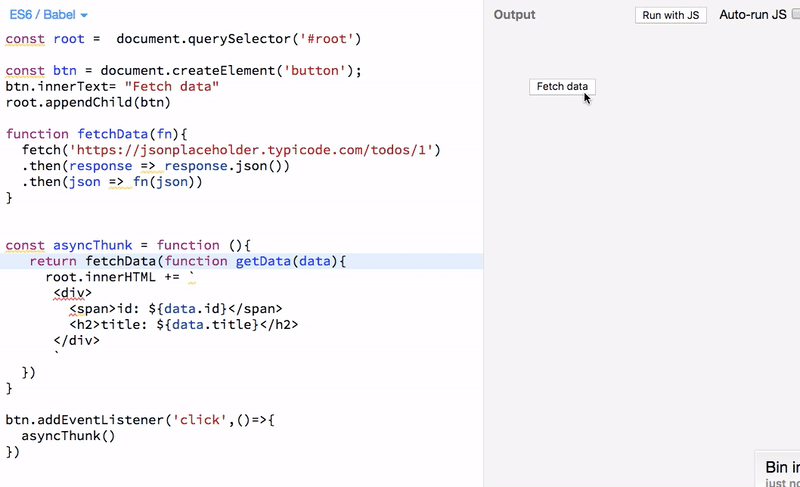# Understanding Thunks in JavaScriptUnderstanding Thunks in JavaScript - In this tutorial, we will learn about what are thunks and how to use the thunks in our JavaScript code.

## Definition of thunk

A thunk is just a function which delays the evaluation of the value.

The thunk function doesn’t take any arguments and gives the value whenever you invoke the thunk.

## Example of synchronous thunk

``````function subtract(x,y){
return x-y;
}

// subtract function is evaluated the
// expression and result is returned immediately
console.log(subtract(5,3)) // 2

// this is a thunk function

// result is delayed untill we call the thunk() function
const thunk = function (){
return subtract(5,3);
}

// we always get the same data whenever we call the thunk function
console.log(thunk()) // 2``````

In the above code, we first defined the subtract function and next we defined thunk function which returns the value of subtract function.

## Asyncrouchronous thunks

Sometimes we need to make network requests in such case we need to use asynchronous thunks.

If you know about redux state management library which uses the asynchronous thunks to delay the actions to dispatch.

Let’s see an example:

``````function fetchData(fn){
setTimeout(()=>fn(1),2000)
}

const asyncThunk = function (){
return fetchData(function(data){
console.log(data)
})
}

asyncThunk()

// 1``````

In the above code, we used setTimeout function to make our code asynchronous.

### Network requests example using thunks

``````function fetchData(fn){
fetch('https://jsonplaceholder.typicode.com/todos/1')
.then(response => response.json())
.then(json => fn(json))
}

const asyncThunk = function (){
return fetchData(function getData(data){
console.log(data)
})
}

asyncThunk()``````

In the above code we are not calling the getData function immediately we only call the getData function whenever the data is available from the API endpoint.In our case, we just logged the data in the console if you want to render the data in the dom you can do it.

## Rendering data exampleIn the above example we used FetchData button to invoke the asyncThunk() function then we render the data in the dom.

## Hire Dedicated eCommerce Web Developers | Top eCommerce Web Designers

Build your eCommerce project by hiring our expert eCommerce Website developers. Our Dedicated Web Designers develop powerful & robust website in a short span of time.